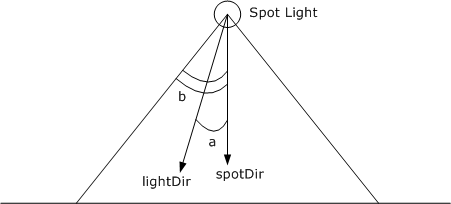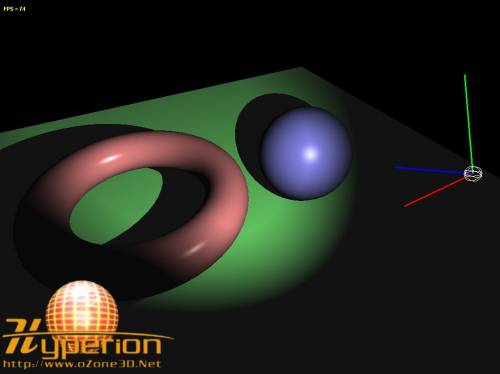Home Utilities Tutorials 3D Demos Graphics Cards Forums About

 GeeXLab
Current version: 0.45.1
>GeeXLab homepage

 FurMark
Current version: 1.30.0
>FurMark homepage

 GPU Caps Viewer
Current version: 1.55.0.0
>GPU Caps Viewer homepage

 GPU Shark
Current version: 0.26.0.0
>GPU Shark homepage

 Blogs
>JeGX's HackLab

 Geeks3D's Articles
>GPU Memory Speed Demystified

>How to Get your Multi-core CPU Busy at 100%

>How To Make a VGA Dummy Plug

>Night Vision Post Processing Filter

 PhysX FluidMark
Current version: 1.5.4
>FluidMark homepage

 TessMark
Current version: 0.3.0
>TessMark homepage

Current version: 0.3.0

 Demoniak3D
Current Version: 1.23.0
>Demoniak3D
>Libraries and Plugins
>Demos
>Codes Samples

3D Graphics Search Engine:Lighting with GLSL Phong Model By Jérôme GUINOT aka 'JeGX' - jegx [at] ozone3d [dot] net Initial draft: February 19, 2006 Update: March 8, 2006 [ Index ]ï¿½Next Page3 - Spot Light in GLSLSpot light radiates its rays in a cone. Shaders codes are the same as for point light but in the pixel shader there is a little change. We add a test in order to check whether or not the light ray direction is located in the cone. To perform this test, we're going to use two GLSL variables: gl_LightSource.spotDirection and gl_LightSource.spotCosCutoff.Fig.2 - Spot Light Configuration.In order to know if the ray of light is located in cone of the light, we're going to compare the angles a and b. If a is smaller than b then the ray is located in the cone. b is the spot cutoff angle (20 degrees in the demo). a is the angle between the direction of the spot and the current processing ray of light. If lightDir and spotDir are normalized vectors, then to calculate a, we can do: dot(lightDir, spotDir) = |lightDir| * |spotDir| * cos(a) dot(lightDir, spotDir) = cos(a) It would be nice to have the possibility to compare cos(a) directly without computing the inverse cosine. That's the role of the variable gl_LightSource.spotCosCutoff. It corresponds to the b angle cosine. Therefore, the test becomes:```if( dot(lightDir, spotDir) > gl_LightSource.spotCosCutoff ) { // Do point light calculations. }```We don't have to forget that the smaller the angle is, the greater the cosine is. That explains the way the test is done. Now, let's see the spot light pixel shader code:```[Pixel_Shader] varying vec3 normal, lightDir, eyeVec; void main (void) { vec4 final_color = (gl_FrontLightModelProduct.sceneColor * gl_FrontMaterial.ambient) + (gl_LightSource.ambient * gl_FrontMaterial.ambient); vec3 L = normalize(lightDir); vec3 L = normalize(lightDir); vec3 D = normalize(gl_LightSource.spotDirection); if (dot(-L, D) > gl_LightSource.spotCosCutoff) { vec3 N = normalize(normal); float lambertTerm = max( dot(N,L), 0.0); if(lambertTerm > 0.0) { final_color += gl_LightSource.diffuse * gl_FrontMaterial.diffuse * lambertTerm; vec3 E = normalize(eyeVec); vec3 R = reflect(-L, N); float specular = pow( max(dot(R, E), 0.0), gl_FrontMaterial.shininess ); final_color += gl_LightSource.specular * gl_FrontMaterial.specular * specular; } } gl_FragColor = final_color; }```The light vector is reversed because defined as the difference between the position of the spot light and the position of the current vertex in processing (see in the vertex shader code). So light and spot direction vectors are in opposition.Fig.3 - spot_light.xml demo.We dare say that the franc limit between the lighted area and the unlighted one is not really realistic. We are going to borrow Direct3D spot lights principle. D3D spots have two cones: the inner cone and the outer cone. The inner cone is equivalent to the OpenGL's one. The purpose is to get a decreasing intensity between the inner and the outer cones in order to create a area of penumbra. In that manner, the edge of the shadow will not be hard anymore but will be gradual and soft. We're going to see a very simple method that consists in decreasing in a linear manner the light intensity using a variable called falloff. This variable is the ratio between the current angle between both cones and the difference between both cones. The shader code will help us to understand the technique:```[Pixel_Shader] varying vec3 normal, lightDir, eyeVec; const float cos_outer_cone_angle = 0.8; // 36 degrees void main (void) { vec4 final_color = (gl_FrontLightModelProduct.sceneColor * gl_FrontMaterial.ambient) + (gl_LightSource.ambient * gl_FrontMaterial.ambient); vec3 L = normalize(lightDir); vec3 D = normalize(gl_LightSource.spotDirection); float cos_cur_angle = dot(-L, D); float cos_inner_cone_angle = gl_LightSource.spotCosCutoff; float cos_inner_minus_outer_angle = cos_inner_cone_angle - cos_outer_cone_angle; if (cos_cur_angle > cos_inner_cone_angle) { vec3 N = normalize(normal); float lambertTerm = max( dot(N,L), 0.0); if(lambertTerm > 0.0) { final_color += gl_LightSource.diffuse * gl_FrontMaterial.diffuse * lambertTerm; vec3 E = normalize(eyeVec); vec3 R = reflect(-L, N); float specular = pow( max(dot(R, E), 0.0), gl_FrontMaterial.shininess ); final_color += gl_LightSource.specular * gl_FrontMaterial.specular * specular; } } else if( cos_cur_angle > cos_outer_cone_angle ) { float falloff = (cos_cur_angle - cos_outer_cone_angle) / cos_inner_minus_outer_angle; vec3 N = normalize(normal); float lambertTerm = max( dot(N,L), 0.0); if(lambertTerm > 0.0) { final_color += gl_LightSource.diffuse * gl_FrontMaterial.diffuse * lambertTerm * falloff; vec3 E = normalize(eyeVec); vec3 R = reflect(-L, N); float specular = pow( max(dot(R, E), 0.0), gl_FrontMaterial.shininess ); final_color += gl_LightSource.specular * gl_FrontMaterial.specular * specular * falloff; } } gl_FragColor = final_color; }```Here is the result:Fig.4 - The spot_light_enhanced_demo.xml demo. Update: March 8, 2006: Here is an optimized version of the previous pixel shader suggested by one of the forum members in this topic This version removes one dynamic branching and seriously improves the code speed (there are about 100 FPS of difference between both pixel shaders!!!):```[Pixel_Shader] varying vec3 normal, lightDir, eyeVec; const float cos_outer_cone_angle = 0.8; // 36 degrees void main (void) { vec4 final_color = (gl_FrontLightModelProduct.sceneColor * gl_FrontMaterial.ambient) + (gl_LightSource.ambient * gl_FrontMaterial.ambient); vec3 L = normalize(lightDir); vec3 D = normalize(gl_LightSource.spotDirection); float cos_cur_angle = dot(-L, D); float cos_inner_cone_angle = gl_LightSource.spotCosCutoff; float cos_inner_minus_outer_angle = cos_inner_cone_angle - cos_outer_cone_angle; //**************************************************** // Don't need dynamic branching at all, precompute // falloff(i will call it spot) float spot = 0.0; spot = clamp((cos_cur_angle - cos_outer_cone_angle) / cos_inner_minus_outer_angle, 0.0, 1.0); //**************************************************** vec3 N = normalize(normal); float lambertTerm = max( dot(N,L), 0.0); if(lambertTerm > 0.0) { final_color += gl_LightSource.diffuse * gl_FrontMaterial.diffuse * lambertTerm * spot; vec3 E = normalize(eyeVec); vec3 R = reflect(-L, N); float specular = pow( max(dot(R, E), 0.0), gl_FrontMaterial.shininess ); final_color += gl_LightSource.specular * gl_FrontMaterial.specular * specular * spot; } gl_FragColor = final_color; }```[ Index ]ï¿½Next Page

 GeeXLab demosGLSL - Mesh exploderPhysX 3 cloth demoNormal visualizer with GS